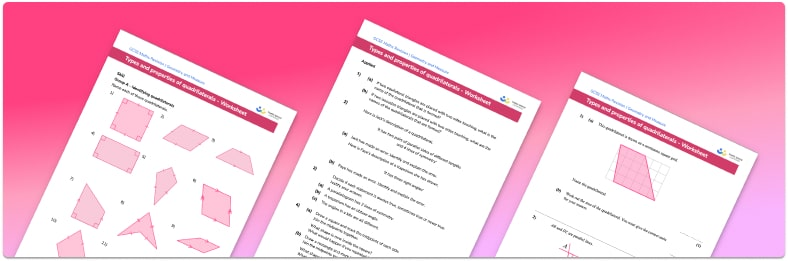# Types And Properties Of Quadrilaterals Worksheet• Section 1 of the types and properties of quadrilaterals worksheet contains 20+ skills-based types and properties of quadrilaterals questions, in 3 groups to support differentiation
• Section 2 contains 3 applied types and properties of quadrilaterals questions with a mix of worded problems and deeper problem solving questions
• Section 3 contains 3 foundation and higher level GCSE exam style types and properties of quadrilaterals questions
• Answers and a mark scheme for all types and properties of quadrilaterals questions are provided
• Questions follow variation theory with plenty of opportunities for students to work independently at their own level
• All questions created by fully qualified expert secondary maths teachers
• Suitable for GCSE maths revision for AQA, OCR and Edexcel exam boards

• This field is for validation purposes and should be left unchanged.

You can unsubscribe at any time (each email we send will contain an easy way to unsubscribe). To find out more about how we use your data, see our privacy policy.

### Types and properties of quadrilaterals at a glance

Quadrilaterals are polygons with four sides. Vertices of a quadrilateral are usually labelled as A B C D. This gives a point of reference for edges and angles within the quadrilateral. There are a number of different types of quadrilaterals we need to know about:

• Square – 4 equal sides, 4 right angles, perpendicular diagonals
• Rectangle – 2 pairs of equal opposite sides, 4 right angles
• Parallelogram – 2 pairs of equal, parallel lines
• Rhombus – 4 equal sides, opposite angles equal, perpendicular diagonals
• Trapezium (Trapezoid) – 1 pair of parallel sides, adjacent angles add up to 180o
• Kite – adjacent sides equal, 1 pair of opposite angles equal, perpendicular diagonals

Looking forward, students can then progress to additional polygons worksheets and other geometry worksheets, for example a simplifying expressions worksheet or simultaneous equations worksheet.For more teaching and learning support on Geometry our GCSE maths lessons provide step by step support for all GCSE maths concepts.

## Do you have KS4 students who need more focused attention to succeed at GCSE?There will be students in your class who require individual attention to help them succeed in their maths GCSEs. In a class of 30, it’s not always easy to provide.

Help your students feel confident with exam-style questions and the strategies they’ll need to answer them correctly with our dedicated GCSE maths revision programme.

Lessons are selected to provide support where each student needs it most, and specially-trained GCSE maths tutors adapt the pitch and pace of each lesson. This ensures a personalised revision programme that raises grades and boosts confidence.

Find out more